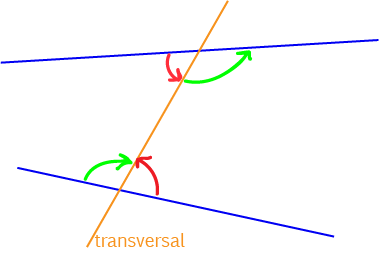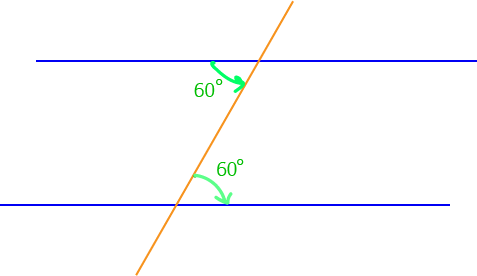# Alternate Angles

A transversal is a line that intersects with two or more other lines.

When transversals cut lines, they form many different pairs of angles. In this article, we're interested in alternate angles, which are sometimes also called alternate interior angles.

## Locating Alternate AnglesIn the picture, the two lines are blue, and the transversal is orange. Alternate angles lie between the two lines, but on either side of the transversal. The picture shows two pairs of alternate angles, one marked by red arrows, and the other marked by green arrows. Can you see how they are the angles inside a "z"-shape? The "z"-shape for the red angles runs along the top blue line from left to right up to the transversal, down the transversal and then from left to right along the bottom blue line. The "z"-shape for the green arrows is back-to-front: from right to left across the top line, down the transversal and from right to left along the bottom blue line.

## Alternate Angles and Parallel Lines

If the transversal cuts a pair of parallel lines, the alternate angles that are formed are equalIn the picture, the two blue lines are parallel, so the transversal forms equal alternate angles. Both alternate angles marked in green are equal to $60^\circ$. They are the angles inside a "z".In the picture, the two blue lines are parallel, so the transversal forms equal alternate angles. Both alternate angles marked in green are equal to $120^\circ$. They are the angles inside a back-to-front "z".In the picture, the two blue lines are parallel, so the transversal forms equal alternate angles. The angles marked in pink are alternate angles, so they must be equal, and $x$ must equal $30^\circ$.

## A Test for Parallel Lines

Two lines, cut by a transversal, are parallel if the alternate angles are equal. So, if I hadn't told you that the two blue lines in this picture were parallel, you could work it out because the two alternate angles marked are equal.Having equal alternate angles is a test for parallel lines. If the alternate angles aren't equal, then the lines aren't parallel. If the alternate angles are equal, then the lines are parallel.

### Description

In this mini book, you will learn about

1. Alternate angles
2. Cointerior angles
4. Vertically Opposite Angles

and several other topics related to lines and angles.

### Audience

Year 10 or higher

### Learning Objectives

To learn the basics of Lines and Angles stream of Geometry

Author: Subject Coach
You must be logged in as Student to ask a Question.